# Test: Single Correct MCQs: The d- and f-Block Elements & Coordination Compounds | JEE Advanced

## 49 Questions MCQ Test Chemistry 35 Years JEE Main & Advanced Past year Papers | Test: Single Correct MCQs: The d- and f-Block Elements & Coordination Compounds | JEE Advanced

Description
Attempt Test: Single Correct MCQs: The d- and f-Block Elements & Coordination Compounds | JEE Advanced | 49 questions in 100 minutes | Mock test for JEE preparation | Free important questions MCQ to study Chemistry 35 Years JEE Main & Advanced Past year Papers for JEE Exam | Download free PDF with solutions
QUESTION: 1

### When same amount of zinc is treated separately with excess of sulphuric acid and excess of sodium hydroxide, the ratio of volume of hydrogen evolved is

Solution: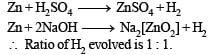QUESTION: 2

### Which of the following is the weakest base

Solution:

∵ Basicity of hydroxides decreases on moving left to right in a period.

QUESTION: 3

### One of the constituent of German silver is

Solution:

German silver is alloy of Cu + Zn + Ni

QUESTION: 4

Which of the following dissolve in hot conc. NaOH solution

Solution: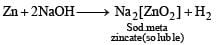QUESTION: 5

How many unpaired electrons are present in Ni2+?

Solution: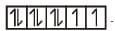It has 2 unpaired electrons. 3d orbital of Ni2+ ion. At No. of Ni = 28.

QUESTION: 6

Sodium thiosulphate is used in photography because of its

Solution:

Hypo solution (Na2S2O3) is used in photography to remove the unaffected AgBr in the form of soluble complex.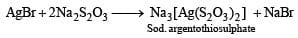QUESTION: 7

Iron is rendered passive by treatment with concentrated

Solution:

Conc. HNO3 renders iron passive by forming a thin protective film of Fe3O4 on its surface.

QUESTION: 8

In the metallurgy of iron, when limestone is added to the blast furnace, the calcium ion ends up in

Solution: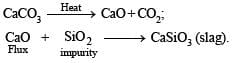QUESTION: 9

Zinc-copper couple that can be used as a reducing agent is obtained by :

Solution:

Zinc-copper couple is obtained by mixing zinc dust with copper guaze.

QUESTION: 10

Amongst the following, the lowest degree of paramagnetism per mole of the compound at 298 K will be shown by

Solution:

Mn 2+ in Mn SO4.4H2O has d 5 con figur ation (five un paired electr on s); Cu2+ in CuSO4.5H2O has d 9 configuration (one unpaired electron); Fe2+ in FeSO4.6H2O has d6 configuration (four unpaired electron); and Ni2+ in NiSO4.6H2O has d8 configuration (two unpaired electron). Thus CuSO4.5H2O has lowest degree of paramagnetism.

QUESTION: 11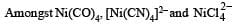Solution: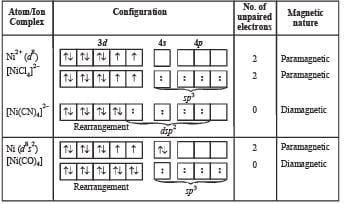QUESTION: 12

Which one is solder ?

Solution:

Solder is an alloy containing Sn – 67% and Pb – 33%.

QUESTION: 13

Which pair gives Cl2 at room temperature?

Solution:

KMnO4 + 16HCl → 2KCl + 2MnCl2 + 8H2O + 3Cl2

QUESTION: 14

Which compound is formed when excess of KCN is added to aqueous solution of copper sulphate?

Solution:

CuSO4 + 2KCN → Cu (CN)2 + K2SO4
2Cu(CN)2 → Cu2(CN)2 +  (CN)2 (Cyanogen)
Cu2(CN)2 + 6 KCN → 2K3 [Cu(CN)4]

QUESTION: 15

Which compound does not dissolve in hot, dilute HNO3?

Solution:

HgS does not dissolved in hot dil. HNO3

QUESTION: 16

An aqueous solution of FeSO4, Al2(SO4)3 and chrome alum is heated with excess of Na2O2 and filtered. The materials obtained are :

Solution:

TIPS/FORMULAE :

Chrome alum is K2SO4.Cr2(SO4)3.24H2O

The filtrate is yellow due to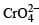ion and residue is brown due to Fe(OH)3.

QUESTION: 17

Ammonium dichromate is used in some fireworks. The green coloured powder blown in the air is

Solution: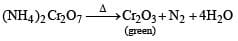Hence green coloured powder blown in the air is Cr2O3.

QUESTION: 18

The number of moles of KMnO4 that will be needed to react with one mole of sulphite ion in acidic solution is

Solution:

The reaction of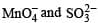in acidic medium is derived as follows: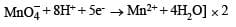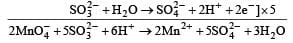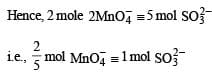QUESTION: 19

Which of the following is an organometallic compound?

Solution:

Organometallic compounds are those compounds in which metal atom is directly bonded with C-atom. H3C – Li.

QUESTION: 20

Which of the following compounds is expected to be coloured?

Solution:

The electronic configurations of cations in the given salts are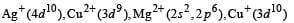Only Cu2+ ion has one unpaired electron in 3d orbital and so, its salt is expected to be coloured.

QUESTION: 21

In the dichromate anion,

Solution:

The structure of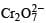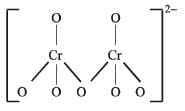There are six normal Cr – O bonds and two bridged Cr – O bonds.
The six normal Cr – O bonds are expected to be equivalent and different from those of the bridged Cr – O bonds.

QUESTION: 22

The geometry of Ni(CO)4 and Ni(PPh3)2Cl2 are

Solution:

NOTE : In metal carbonyl the metal is in zero oxidation state.
In Ni(CO)4 , O.N. of Ni = 0
For Ni (Z = 28)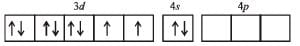In presence of CO two 4s electrons pair up, thus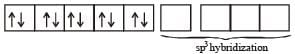In Ni(PPh3)2Cl2, O.N. of Ni = +2 For Ni2+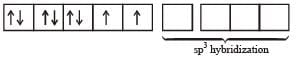PPh3 and Cl can’t pair up d-electrons, leading to sp3 hybridization leading to tetrahedral geometry.

QUESTION: 23

The chemical processes in the production of steel from haematite ore involve

Solution:

Haematite ore (Fe2O3) is first reduced to cast iron which is then oxidised for removing carbon (impurity) as CO2.

QUESTION: 24

The complex ion which has no ‘d’ electron in the central metal atom is

Solution:

In [MnO4], Mn is in +7 oxidation state.
Electronic configuration of Mn (Z = 25) : [Ar] 3d54s2
Electronic configuration of Mn7+  : [Ar] 3d04s0 Central atom in other ions have definite number of d electrons
No. of electrons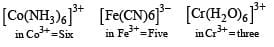QUESTION: 25

Anhydrous ferric chloride is prepared by

Solution:

2Fe + 3Cl2 (dry) → 2FeCl3 (anhydrous)

QUESTION: 26

When MnO2 is fused with KOH, a coloured compound is formed, the product and its colour is:

Solution:

Stable oxidation state of Mn in alkaline medium is +6.
So, MnO2 is oxidised to K2MnO4 (purple green) by atmospheric oxygen in KOH medium.QUESTION: 27

In the process of extraction of gold,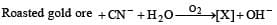[X ] + Zn → [Y] + Au

Identify the complexes [X] and [Y]

Solution: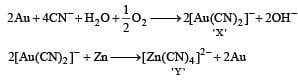QUESTION: 28

The species having tetrahedral shape is

Solution:

The configuration of Ni2+ is 3d8. For the elements of the first transition series, Cl behaves as a weak field/ high spin ligand. Hence Ni in [NiCl4]2– is sp3  hybridised leading to tetrahedral shape.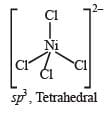QUESTION: 29

The spin magnetic moment of cobalt in the compound Hg[Co(SCN)4] is

Solution:

TIPS/FORMULAE :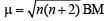(m= spin magnetic moment) Here Co is present as Co2+ ion which has 3 unpaired electrons. So the spin magnetic moment will be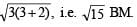QUESTION: 30

The product of oxidation of I with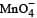in alkaline medium is

Solution: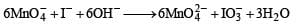QUESTION: 31

(NH4)2Cr2O7 on heating liberates a gas. The same gas will be obtained by

Solution: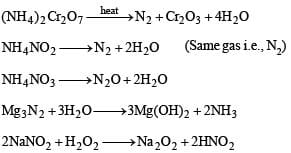QUESTION: 32

Which pair of compounds is expected to show similar colour in aqueous medium?

Solution:

Colour of transition metal ion salt is due to d-d transition of unpaired electrons of d-orbital. Metal ion salt having similar number of unpaired electrons in d-orbitals shows similar colour in aqueous medium.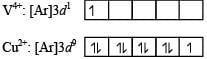QUESTION: 33

Which kind of isomerism is exhibited by octah edral Co(NH3)4Br2Cl?

Solution:

Co(NH3)4Br 2Cl will show both geometr ical a n d ionization isomerism. [Co(NH3)4Br2]Cl and [Co(NH3)4BrCl]Br are ionization isomers and geometrical isomers are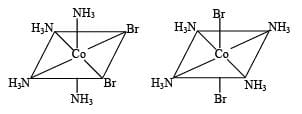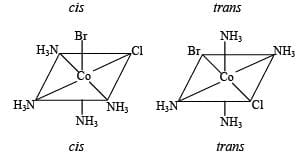QUESTION: 34

CuSO4 decolourises on addition of KCN, the product formed is

Solution: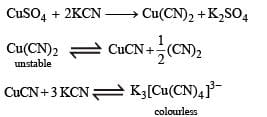QUESTION: 35

Among the following metal carbonyls, the C–O bond order is lowest in

Solution:

An anionic carbonyl complex can delocalise more electron density to antibonding pi-orbital of CO and hence, lowers the bond order.

QUESTION: 36

Native silver metal forms a water soluble complex with a dilute aqueous solution of NaCN in the presence of

Solution:

In the presence of oxygen, Ag metal forms a water soluble complex Na [Ag (CN)2] with dilute solution of NaCN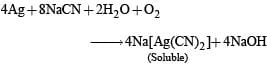QUESTION: 37

Among the following, the coloured compound is

Solution:

NOTE :  Colour is due to d – d transitions. Coloured compounds contain partly filled d-orbital.
The oxidation state of copper in various compounds is + 1 and + 2. In CuF2 it is in + 2 oxidation state. In + 2 state its configuration is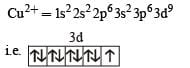It has one unpaired electron due to this it is coloured.
The colour is due to d-d transitions. (NOTE : CuF2 possesses blue colour in crystalline form)

QUESTION: 38

The IUPAC name of [Ni (NH3)4] [NiCl4] is

Solution:

The correct IUPAC name of the given compound is tetramminenickel (II) - tetrachloronickelate (II) thus (c) is the correct answer.

QUESTION: 39

Both [Ni (CO)4] and [Ni (CN)4]2– are diamagnetic. The hybridisations of nickel in these complexes, respectively, are

Solution:

NOTE : : In carbonyls O.S. of metal is zero In [Ni(CO)4], the oxidation state of nickel is zero. Its configuration in Ni(CO)4 is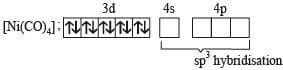In [Ni(CN)4]2– the oxidation state of Ni is 2+ and its configuration is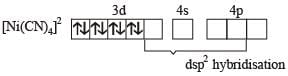Thus the hybridisations of nickel in these compounds are sp3 and dsp2 respectively.
Hence (b) is the correct answer.

QUESTION: 40

The spin only magnetic moment value (in Bohr magneton units) of Cr(CO)6 is

Solution:

Chromium in Cr (CO)6 is in zero oxidation state and has [Ar]18 3d54s1 as the electronic configuration. However, CO is a strong ligand, hence pairing up of electrons takes place leading to following configuration in Cr(CO)6.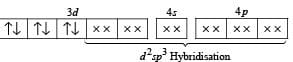Since the complex has no unpaired electron, its magnetic moment is zero.

QUESTION: 41

The ionisation isomer of [Cr(H2O)4Cl(NO2)]Cl is

Solution:

Ionisation isomer of [Cr(H2O)4 Cl (NO2)]Cl is [Cr(H2O)4Cl2]NO2.

QUESTION: 42

The correct structure of ethylenediaminetetraacetic acid (EDTA) is

Solution:

The correct structure of EDTA is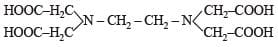QUESTION: 43

The complex showing a spin-only magnetic moment of 2.82 B.M. is :

Solution: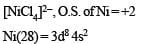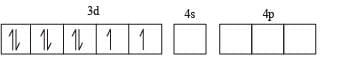Cl being weak ligand it cannot pair up the two electrons present in 3d orbital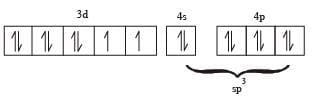No. of unpaired electrons = 2 Magnetic moment, μ = 2.82 BM.

QUESTION: 44

Among the following complexes (K-P)

K3[Fe(CN)6] (K), [Co(NH3)6]Cl3(L), Na3[Co(oxalate)3] (M), the [Ni(H2O)6]Cl2(N), K2[Pt(CN)4] (O) and  [Zn(H2O)6](NO3)2(P) the diamagnetic complexes are

Solution: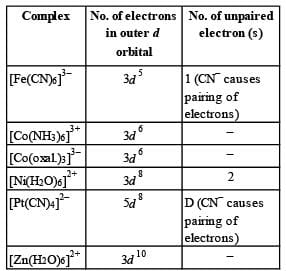Thus L, M, O and P are diamagnetic.

QUESTION: 45

As per IUPAC nomenclature, the name of the complex [Co(H2O)4(NH3)2]Cl3 is :

Solution:

[Co(H2O)4(NH3)2]Cl3
= Diamminetetraaquacobalt (III) chloride.

QUESTION: 46

The colour of light absorbed by an aqueous solution of CuSO4 is:

Solution:

CuSO4 will be absorbing orange-red colour and hence will be of blue colour.

QUESTION: 47

NiCl2 {P(C2H5)2(C6H5)}2 exhibits temperature depend-ent magnetic behaviour (paramagnetic/diamagnetic). The coordination geometries of Ni2+ in the paramagnetic and diamagnetic states are respectively

Solution:

In both states (paramagnetic  and diamagnetic) of the given complex, Ni exists as Ni2+ whose electronic configuration is [Ar] 3d84s0.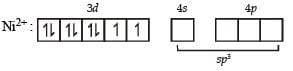In the above paramagnetic state the geometry of the complex is sp3 giving tetrahedral geometry.
The diamagnetic state is achieved by pairing of electrons in 3d orbital.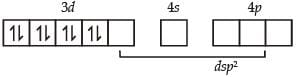Thus the geometry of the complex will be dsp2 giving square planar geometry.

QUESTION: 48

Consider the following complex ions, P, Q and R.

P = [FeF6]3–, Q = [V(H2O)6]2+ and R = [Fe(H2O)6]2+

The correct order of the complex ions, according to their spin-only magnetic moment values (in B.M.) is

Solution:

The electronic configuration of central metal ion in complex ions P, Q and R are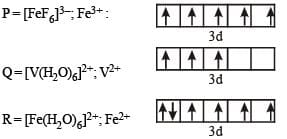Higher the no. of unpaired electron(s), higher will be magnetic moment.
Thus the correct order of spin only magnetic moment is  Q < R < P

QUESTION: 49

Among [Ni(CO)4], [NiCl4]2– , [Co(NH3)4Cl2]Cl, Na3[CoF6], Na2O2 and CsO2, the total number of paramagnetic compounds is

Solution: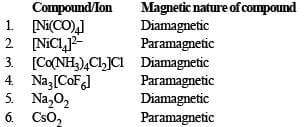So total number of paramagnetic compounds is 3.Use Code STAYHOME200 and get INR 200 additional OFF Use Coupon Code